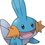# This Set

Summary:

Do you want to practice for an Olympiad? Or perhaps you just got bored and (coincidentally) saw this? Whatever the reason may be, I hope you would try this out!

How it started:

I was recently in at least $2$ contests this year, excluding my training. I was quite bummed out because I solve problems too slowly. If I do it fast, I will get a ton of careless mistakes. If I do it slow, I wouldn't finish the test in time. So I made these sample tests and I test myself a test every week. This is one of my sample tests just so that you will know how I do things here. Have fun!

This is like a test I do every week or so. I will collect $15$ to $16$ problems ranging from Level $3$ to Level $5$ and I will time myself for $4$ to $5$ hours. Depending on the difficulty (I can estimate their difficulties by looking at their levels).

Once you are done answering, compile all your answers and solutions. You should not change your answers anymore. Afterwards, input all you answers into the problem in here (Brilliant). In case your answer is wrong, even due to arithmetic errors, that is still considered as a mistake in the test, but you may input another answer in Brilliant after you have re-checked things.

And in case you have answered some problems already, try to shorten the time then. :P

If you would ask why they're problems made by other people, I do that to be fair. Because if that problem was posted by me, then I would already know the answer.

<Edit> I excluded $B^2$ problems because some of my friends don't have $B^2$ accounts.

For this test, there will be $16$ problems and a time limit of $5$ hours will be given. Good Luck! :DNote by Sean Ty
6 years, 10 months ago

This discussion board is a place to discuss our Daily Challenges and the math and science related to those challenges. Explanations are more than just a solution — they should explain the steps and thinking strategies that you used to obtain the solution. Comments should further the discussion of math and science.

When posting on Brilliant:

• Use the emojis to react to an explanation, whether you're congratulating a job well done , or just really confused .
• Ask specific questions about the challenge or the steps in somebody's explanation. Well-posed questions can add a lot to the discussion, but posting "I don't understand!" doesn't help anyone.
• Try to contribute something new to the discussion, whether it is an extension, generalization or other idea related to the challenge.

MarkdownAppears as
*italics* or _italics_ italics
**bold** or __bold__ bold
- bulleted- list
• bulleted
• list
1. numbered2. list
1. numbered
2. list
Note: you must add a full line of space before and after lists for them to show up correctly
paragraph 1paragraph 2

paragraph 1

paragraph 2

[example link](https://brilliant.org)example link
> This is a quote
This is a quote
    # I indented these lines
# 4 spaces, and now they show
# up as a code block.

print "hello world"
# I indented these lines
# 4 spaces, and now they show
# up as a code block.

print "hello world"
MathAppears as
Remember to wrap math in $$ ... $$ or $ ... $ to ensure proper formatting.
2 \times 3 $2 \times 3$
2^{34} $2^{34}$
a_{i-1} $a_{i-1}$
\frac{2}{3} $\frac{2}{3}$
\sqrt{2} $\sqrt{2}$
\sum_{i=1}^3 $\sum_{i=1}^3$
\sin \theta $\sin \theta$
\boxed{123} $\boxed{123}$

Sort by:

Great Idea!

Staff - 6 years, 10 months ago

Thank you Mr. Calvin! :D

- 6 years, 10 months ago